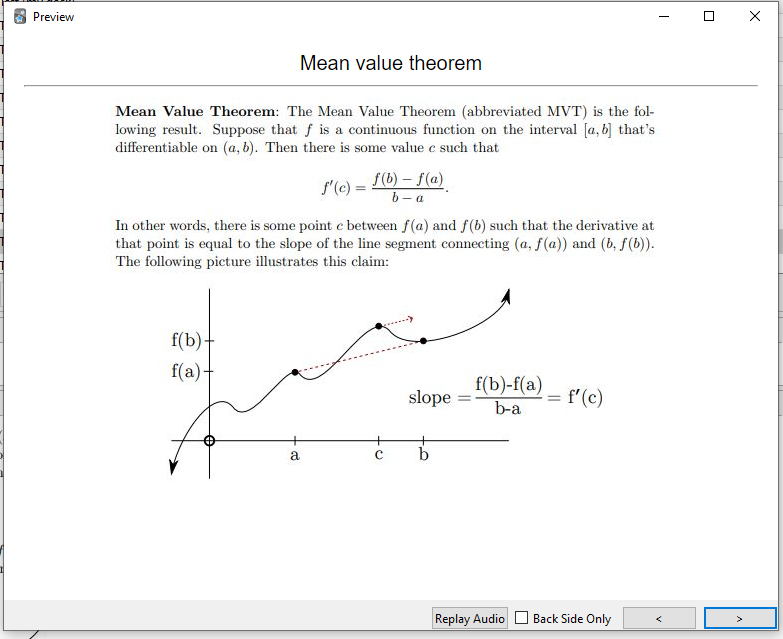# Blog

## GRE Math Subject Test

### 10 Jul 2022

My blog has been pretty quiet recently, because I’ve been focusing on studying for the GRE Mathematics Subject Test. I think this test is sort of unique among popular, standardized tests, because the problems on it are very difficult. There are 66 questions on each test, and it must be done in 2 hours and 50 minutes. That means the test-taker has 2 minutes and 35 seconds to answer questions like these:

Of the following, what is the best approximation of $$\sqrt{1.5}(266)^{3/2}$$?

(A) 1,000 (B) 2,700 (C) 3,200 (D) 4,100 (E) 5,300

What is the units digit in the standard decimal expansion of the number $$7^{25}$$?

(A) 1 (B) 3 (C) 5 (D) 7 (E) 9

Let $$V$$ be the real vector space of all real $$2\times 3$$ matrices, and let $$W$$ be the real vector space of all $$4\times 1$$ column vectors. If $$T$$ is a linear transformation from $$V$$ onto $$W$$, what is the dimension of the subspace $${\mathbf{v}\in V: T(\mathbf{v}) = \mathbf{0}}$$?

(A) 2 (B) 3 (C) 4 (D) 5 (E) 6

Calculators are not allowed. Of course, none of these questions are as hard as they look, you just have to know the central theorem or trick. Still, the test is very tough. I personally know the price of underestimating the subject test. When I took it in my senior year of college, I didn’t really study and got an unimpressive score. I thought my proficiency in math from classes and my research would be enough, and it wasn’t.

I wanted to put some of the resources that I’m using online, so other people can use them if they want.

First, I’ve got my Anki deck. Anki is a flashcard program which uses spaced repetition to schedule flashcards based on their difficulty. It’s really useful for basic memorization. I encourage whoever uses the deck to not just memorize the theorems, but also develop an understanding of what they look like “under the hood” – the related proofs and intuition. But I also challenge the idea that a good mathematician never has to memorize things, and for a test where time is a major factor, I think it’s mandatory to have these things available for quick recall. Here’s one of the cards:For textbooks, I’ve got a copy of Stewart’s Calculus: Early Transcendentals. This is probably the most important one, since the test if 50% calculus problems of various difficulty. I also have Rudin’s Principles of Mathematical Analysis, which is basically a real analysis bible. For linear algebra, I have Linear Algebra Done Right by Sheldon Axler. For abstract algebra, I have Abstract Algebra by Tom Judson, which is available freely online! For topology, I have Elementary Topology by Michael Gemignani.

There are a lot of great resources online, as well. UChicago has a great website where they have 5 previous GREs, with answer keys and a scoring guide. They also have 6 locally generated practice tests, which are a fair bit harder than the average GRE. Ian Coley at Rutgers has written full solutions to the available GRE tests. He also has a set of lecture notes for a “GRE bootcamp,” with some solutions to common problems. There are a lot of other practice tests floating around out there, most of which are linked at the UChicago site.

I take the exam in September. I’ll let you know how I do!